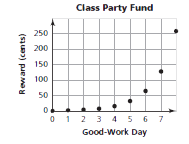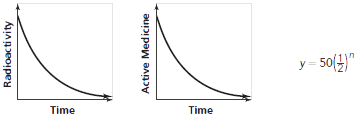Toggle Accessibility Tools

# 8-3 Growing, Growing, Growing - Concepts and Explanations

## Exponential Growth

An exponential pattern of change can often be recognized in a verbal description of a situation or in the pattern of change in a table of (x, y) values.

The exponential growth in rewards for good-work days in the example can be represented in a graph. The increasing rate of growth is reflected in the upward curve of the plotted points.

### ExampleSuppose a reward is offered for days of good work. At the start, 1¢ is put in a party fund. On the first good-work day, 2. is added; on the second good-work day, 4. is added; and on each succeeding good-work day, the reward is doubled. How much money is added on the eighth good-work day?

Good-Work Day Reward (cents)
0 (start) 1
1 2
2 4
3 8
4 16
5 32
6 64
7 128
8

## Growth Factor

A constant factor can be obtained by dividing each successive y-value by the previous y-value. This ratio is called the growth factor of the pattern.

### Example

For each good-work day, the reward doubles. You multiply the previous award by 2 to get the new reward. This constant factor can also be obtained by dividing successive y-values: 2/1 = 2, 4/2 = 2, etc

## Exponential Equation

Examining the growth pattern leads to a generalization that can be expressed as an equation.

An exponential growth pattern y = a(b)x may increase slowly at first but grows at an increasing rate because its growth is multiplicative. The growth factor is b.

### Example

Day Calculation Reward (cents)
0 1 1
1 1 x 2 = 21 2
2 1 x 2 x 2 = 22 4
3 1 x 2 x 2 x 2 = 23 8
: : :
6 1 x 2 x 2 x 2 x 2 x 2 x 2 = 26 64
: : :
n 1 x 2 x 2 x ... x 2 = 2n 2n

On the nth day, the reward R will be R = 1 x 2n. Because the independent variable in this pattern appears as an exponent, the growth pattern is called exponential. The growth factor is the base 2. The exponent n tells the number of times the 2 is a factor.

## Exponential Decay

Exponential models describe patterns in which the value decreases. Decay factors result in decreasing relationships because they are less than 1.

### Example## Rules of Exponents

Students begin to develop understanding for the rules of exponents by examining patterns in a powers table for the first 10 whole numbers.

### Example

By examining the multiplicative structure of the bases:

82 = (2 x 2 x 2)2 = (23)2 = 26; the general pattern is (bm)n = bmn

9 x 27 = 243 or 32 x 33 = 35; in general, (bm)(bn) = bm+n

4 x 25 = 22 x 52 = (2 x 5)2 = 102 = 100; in general, (ambm) = (ab)m

Similar explorations lead to the rule am/an = am-n.